# Binary De Bruijn sequences for DS-CDMA systems: analysis and results

## Introduction

As a consequence, the spreading sequence allocated to each user is an essential element in the design of any CDMA system, as it provides the signal with the requested coded format, and ensures the necessary channel separation mechanism. As in any multi-user communication technique, mutual interference among active users is inherent to a CDMA scheme, and, again, it may be strongly affected by the periodic and non-periodic cross-correlation properties of the whole set of spreading codes selected for adoption . Further, the number of active users and their relative power levels also affect the performance of a CDMA system, besides the propagation channel conditions. But when the number of active users is fixed, and a specific channel scenario is considered, it is possible to investigate the performance of a CDMA system as a function of the properties exhibited by the spreading codes chosen. Bounds on the system performance are determined by the type of codes used, their length, and their chip rate, and may be changed by selecting a different code set.

Several families of codes have been traditionally adopted for spread spectrum purposes, such as Maximal-length sequences (m-sequences), Gold, and Kasami sequences. Either Gold or Kasami sequences are derived by means of well-known algorithms from m-sequences that are generated through Linear Feedback Shift Registers (LFSRs) and exhibit a number of interesting features. In the context of CDMA systems, the most remarkable property is the two valued auto-correlation profile provided by an m-sequence that allows for a precise synchronization of each user at the receiver. Gold and Kasami sequences are mostly valued for the cardinality of their sets, and for the favorable cross-correlation properties they provide that are necessary to ensure as limited interference as possible . Orthogonal variable spreading factor (OVSF) codes  are adopted in Wideband CDMA as channelization codes, thanks to the orthogonality ensured by codes belonging to the same set, i.e., at a parity of their Spreading Factor (SF). OVSF codes may show very differentiated correlation properties, and do not ensure orthogonality when used asynchronously. This article focuses on the evaluation of a class of binary sequences, named De Bruijn sequences that have been studied for many years , but not considered, at the authors' best knowledge, in the framework of multi-user communication systems, as a candidate family of spreading codes to apply. Binary De Bruijn sequences are a special class of nonlinear shift register sequences with full period L = 2n: n is called the span of the sequence, i.e., the sequence may be generated by an n-stage shift register. In the binary case, the total number of distinct sequences of span n is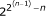; in the more general case of span n sequences over an alphabet of cardinality, α, the number of distinct sequences is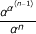. In this article, we refer to binary De Bruijn sequences. The construction of De Bruijn sequences has been extensively investigated, and several different generation techniques have been proposed in the literature [10, 11]; however, due to the exceptional cardinality of their sets, the exhaustive generation of De Bruijn sequences of increasing length is still an open issue. The doubly exponential number of sequences is also a major impediment to characterizing the entire sequence family. At the same time, cardinality is one of the most valued properties of De Bruijn sequences, especially in specific application contexts such as cryptography; on the other hand, not so much is known about the correlation features of the sequences. If adequate, it would be possible to adopt De Bruijn sequences to implement a DS-CDMA communication system, thanks to the huge number of different users that could share the radio channel.

In this article, we investigate the possibility of using binary De Bruijn sequences as spreading codes in DS-CDMA systems, by studying the correlation properties of such sequences and extending the preliminary results presented in . Given the amount of binary De Bruijn sequences obtainable, even for small values of the span parameter, and considering the great complexity of the generation process , we can provide an exhaustive analysis of binary sequences of length 32 (i.e., span 5) that form a set of 2,048 different sequences, and partial results for sequences generated by increasing values of the span.

The article is organized as follows: section "System model" provides a basic description of the DS-CDMA reference model adopted in the paper; section "Binary De Bruijn sequences and their correlation properties" discusses the main properties of binary De Bruijn sequences, with a specific focus on the properties considered relevant to our context. Section "Evaluation of binary De Bruijn sequences in DS-CDMA systems" evaluates the applicability of De Bruijn sequences in DS-CDMA by providing several results obtained through simulations; finally, the article concludes.

## System Model

### DS-CDMA fundamentals

The basic theory of DS-CDMA is well known: the main principle is to spread the user information, i.e., data symbols, by a spreading sequence c(k)(t) of length L. The development of the theoretical model shows that several terms may affect symbol estimation: the desired signal of the k th user, the multiple access interference, the additive noise, and the multipath propagation effect. Due to the multiple access interference term, information bit estimation may be wrong with a certain probability, even at high signal-to-noise ratio (SNR) values, leading to the well-known error-floor in the BER curves of DS-CDMA systems.

Phase-coded spread spectrum multiple access systems, such as DS-CDMA, may be analyzed by modelling phase shifts, time delays, and data symbols as mutually independent random variables (Pursley et al., ). Interference terms are random as well, and treated as additional noise. By this way, the SNR at the output of a correlation receiver in the system is computed by means of probabilistic expectations, with respect to the phase shifts, time delays, and data symbols. According to such an approach, in asynchronous DS-CDMA systems, the average interference parameter may be expressed by: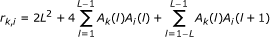(1)

where A k (l) denotes the aperiodic correlation function of the k th user's spreading sequence c(k)(t), with period L. The aperiodic correlation function is, in its turn, defined as: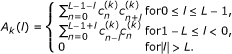(2)

The average SNR at the output of a correlator receiver of the i th user among the K users in the system, under AWGN environment, is given by: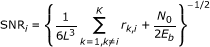(3)

and the average bit error probability for the i th user is defined as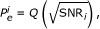(4)

provided a Gaussian distribution for the MAI term, and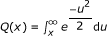. According to Equation 3, the signal-to-noise ratio of the i th user in the system can be evaluated without knowledge of the cross-correlation functions of the spreading codes used, but by resorting to the proper aperiodic correlation definition. When dealing with binary De Bruijn sequences, avoiding the need to exhaustively evaluate the cross-correlation values in a given family may be very important, due to the computational burden associated to the huge cardinality of a set. In any case, cross-correlation between sequences is equally significant in multi-user communication systems, because it is a measure of the agreement between different codes, i.e., of the channel separation capability. The same family of spreading codes may provide very different performances when evaluating their auto- or cross-correlation. As an example, the m-sequences themselves, though providing optimal auto-correlation, are not immune to cross-correlation problems and may have large cross-correlation values. In , Welch obtained a lower bound on the cross-correlation between any pair of binary sequences of period L in a set of M sequences, given by: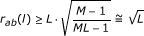(5)

where a and b are two binary sequences in the set having the same period L, and l denotes any possible value of the shift among the sequences (0 ≤ lL - 1); the approximation holds when M L (increasing value of the span n). It is shown in the following that the approximation is tightly verified by De Bruijn binary sequences, due to the double exponential growth of M with n they feature. Being Equation 5 a lower bound, it may help in identifying the sequences showing the worst behavior, i.e., those providing the highest value of the bound.

In the following, we will provide discussions about the correlation properties of binary De Bruijn sequences, that represent the specific set of full-length sequences we are interested in. In section "Evaluation of binary De Bruijn sequences in DS-CDMA systems," a comparative evaluation of the Welch bound for different families of binary spreading codes will be also presented.

### Channel model

In order to test the performance obtainable by the application of De Bruijn sequences as spreading codes in a classical DS-CDMA system, we assume a gaussian channel affected by multipath that is described by means of either the indoor office test environment and the outdoor to indoor and pedestrian test environment described in . In both the cases, the so-called Channel A specified by the Recommendation has been considered.

Both the channel configurations are simulated by means of a tapped-delay-line model, with different values assigned to relative delay (in ns) and average power (in dB) of each path: there are five secondary paths in the indoor test environment, and three secondary paths in the outdoor model. A detailed description of each model may be found in the related reference. Such channel models have been taken as a reference to test the performance of a DS-CDMA system when different choices of the spreading codes are performed, as discussed in section "Evaluation of binary De Bruijn sequences in DS-CDMA systems."

## Binary De Bruijn Sequences and their Correlation Properties

The states S0, S1, . . ., SN - 1of a span n De Bruijn sequence are exactly 2n different binary n-tuples; when viewed cyclically, a De Bruijn sequence of length 2n contains each binary n-tuple exactly once over a period. Being maximal period binary sequences, the length of a De Bruijn sequence is always an even number.

When comparing the total number of De Bruijn sequences of length L to the total number of available m-sequences, Gold, or Kasami sequences, similar but not identical length values shall be considered, as reported in Table 1. The table confirms the double exponential growth in the cardinality of De Bruijn sequences, at a parity of the span n, with respect to the other sequences. Of course, not all the De Bruijn sequences of span n may be suitable for application in a multi-user system; anyway, even if strict selection criteria are applied, it is reasonable to expect that a quite extended subset of sequences may be extracted from the entire family.

About the auto-correlation values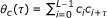, τ = 0, 1, . . ., L - 1, assumed by a De Bruijn sequence c of span n and period L, for a given shift τ, the known results are as follows: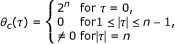(6)

Further, for n ≥ 3, θ c (2n-1) is a multiple of 8.

The second property implies that as long as the span of the sequence increases, there exist more values of the shift τ for which the auto-correlation sidelobes (i.e., the values assumed for τ ≠ 0) are zero. Obviously, at a parity of the chip time, the time duration of each null sample is reduces. These null values are adjacent to the auto-correlation peak value, and contribute to provide resistance against possible multipath effects. It may be shown that the auto-correlation profile is always symmetric with respect to the central value of the shift, and that θ c (τ) ≡ 0 mod 4 for all τ, for any binary sequence of period L = 2n, with n ≥ 2. As any binary De Bruijn sequence c comprises the same number of 1's and 0's, when converted into a bipolar form, the following holds: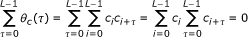(7)

So, when n increases, the auto-correlation profiles of the De Bruijn sequences will show many samples equal to 0, a symmetric distribution of the samples, and a reduced number of different positive and negative samples, as to give an average auto-correlation equal to 0. Figure 1 shows the average auto-correlation profile of the set of span 5 De Bruijn sequences that confirms the previous properties.

A simple bound may be defined for the positive values of the correlation functions sidelobes in De Bruijn sequences :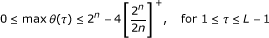(8)

where [x]+ denotes the smallest integer greater than or equal to x. The left inequality follows from the second and the third properties in (6); the right inequality is due to the peculiar features of De Bruijn sequences that are full-length sequences, a period of which includes all the possible binary n-tuples. In the case of binary De Bruijn sequences of span n = 5, the bound gives 0 ≤ maxθ (τ) ≤ 16.

The cross-correlation computed between pairs of De Bruijn sequences a and b randomly chosen, of the same span and period L, denoted as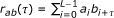, for 0 ≤ τL - 1, exhibits properties very similar to those discussed for the auto-correlation function: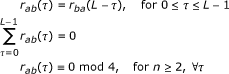For the cross-correlation function of a pair of De Bruijn sequences a and b (ab) of the same span n, the following bound holds :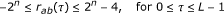(9)

All the possible cross-correlation values are integer multiple of 4. Figure 2 shows the average cross-correlation profile of binary De Bruijn sequences of span 5.

It is worth noting that De Bruijn sequences may be piecewise orthogonal, meaning that it is possible to find two sequences having null cross-correlation for several values of the shift parameter τ. On the other hand, it is also possible that two De Bruijn sequences have an absolute value of the cross-correlation equal to 2n for some value of the shift τ (e.g. complementary sequences), as stated by the bound equation above. This variability in the cross-correlation behavior of the sequences may affect the performance of the CDMA system, when the spreading sequences associated to each user are chosen randomly from the whole set; it will be discussed in the following, with reference to the case of span n = 5 sequences. This also motivates the need for a proper selection criterion to be applied on the whole set of sequences, to extract the most suitable spreading codes to use in the DS-CDMA system.

## Evaluation of Binary De Bruijn Sequences in DS-CDMA Systems

As previously stated in the "Introduction," we can provide a comprehensive evaluation of binary De Bruijn sequences of length 32, i.e., n = 5, which form a set of 2,048 different sequences because, given the small value of the span parameter considered, it is possible to generate the whole set of sequences by means of an exhaustive approach, which may be intended as a brute force one: all the possible binary sequences of length 2n are generated, then the ones satisfying the De Bruijn definition are selected.

For increasing values of n, the brute force generation process becomes unfeasible, and more sophisticated techniques shall be applied . A useful overview of possible alternative approaches suggested in the literature may be found in . However, the main limitation of such solutions is related to the reduced number of sequences they allow to obtain by a single generation step. As a consequence, in this article, we opted for a generation strategy that we named "tree approach". Basically, sequence generation starts with n zeros (the all-zero n-tuple shall be always included in a period of a span n De Bruijn sequence) and appends a one or a zero, as the next bit of the sequence, thus originating two branches. As long as the last n-tuple in the partial sequence obtained has not yet appeared before, generation goes on by iterating the process; otherwise the generation path is discarded. This generation scheme that proceeds by parallel branches is fast to execute, and has the advantage of providing the whole set of sequences that we need to perform our correlation-related evaluations. However, the approach suggested suffers for memory limitations, because all the sequences having the same span n must be generated at the same time. As a consequence, taking into account our focus on the correlation properties of the sequences, we introduce in the generation process a constraint related to cross-correlation: when two generation paths share a common pattern of bits in their initial root, one of them is pruned, in order to reduce a priori the number of sequences that will provide high cross-correlation, due to the presence of common bit patterns.

Before moving to the evaluation of the auto- and cross-correlation properties of binary De Bruijn sequences, for n = 5 and n = 6, let us compare the behavior of such sequences to other families of spreading codes, with respect to the Welch bound discussed above.

### De Bruijn sequences and the Welch bound

As previously stated, the Welch bound allows to evaluate a family of binary spreading codes in terms of its cross-correlation performance. The bound is a lower one, as a consequence, by evaluating such bound over different code sets we can draw conclusions about the one providing the worst performance, i.e., the one for which the bound assumes the highest value. According to this statement, we can compare the Welch bound profile of different sets of spreading codes, namely m-sequences, Gold, OVSF, Kasami, and De Bruijn sequences, at a parity of the span n. To such an aim, we first compute the expression of the Welch bound for each set of spreading codes, starting from the general definition of Equation 5. In the case of OVSF sequences, we assume even values of the spreading factor, given by SF = 2n.

#### Welch bound for m-sequences

The number of m-sequences of period L = 2n - 1 is given by the number of primitive polynomials of degree n, i.e., ϕ (L)/n, where ϕ is the Euler's totient function . So we have M = ϕ (L)/n and, by substitution into Equation 5, we get: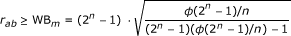(10)

where WB m denotes the expression of the Welch bound for m-sequences.

#### Welch bound for Gold sequences

Gold sequences are generated from the so-called preferred pairs of m-sequences, for values of the span n that satisfy the conditions: n ≠ 0 (mod 4) or n = 2 (mod 4). In the case of Gold sequences, we have L = 2n - 1, and M = L + 2 = 2n + 1, so that: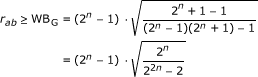(11)

#### Welch bound for OVSF sequences

OVSF sequences are adopted as channelization codes in Wideband CDMA (WCDMA), together with Gold codes used as information scrambling sequences. The main feature of OVSF codes that are derived from Walsh-Hadamard sequences is to be mutually orthogonal at a parity of the SF parameter. However, the orthogonality is ensured in the synchronous case, whereas it is usually lost when OVSF codes are applied asynchronously. Codes in the same OVSF family may exhibit different autocorrelation behaviors, with the possible presence of autocorrelation peaks even for values of the shift τ ≠ 0. The cross-correlation function is zero for OVSF codes of the same SF, and not null in the other cases. In the case of OVSF sequences we have L = M = SF , so that: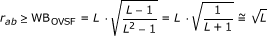(12)

When the SF is a power of 2, as in the simulated cases, we can express it as SF = 2n so that r ab WBOVSF 2n/2. However, it is worth noting that in the specific case of OVSF sequences, for which M = L, the condition of validity of the Welch bound approximation is not strictly verified.

#### Welch bound for Kasami sequences

In the case of Kasami sequences that are generated from m-sequences as well, we have to distinguish between the so-called small set and the large set of sequences. A procedure similar to that used to generate Gold codes permits to obtain the small set of Kasami sequences, that have M = 2n/2and a period L = 2n - 1, where n is even. The large set of Kasami sequences contains, again, sequences of period L = 2n - 1 for n even, and includes either the Gold sequences or the small set. For this set, we have M = 23n/2if n = 0 (mod 4), and M = 23n/2+ 2n/2if n = 2 (mod 4).

So, for the small set of Kasami sequences, when n is even: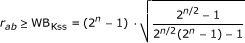(13)

For the large set of Kasami sequences: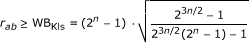(14)

when n = 0 (mod 4), and: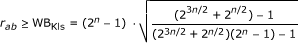(15)

when n = 2 (mod 4).

#### Welch bound for De Bruijn sequences

In the case of binary De Bruijn sequence, for any value of the span n we have: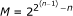and L = 2n , so that: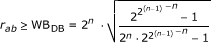(16)

Once derived the expression of the Welch bound specific for each code set, it is possible to compare the sequences' behaviors by evaluating each bound equation for different values of the span n, ranging from 3 to 10. Figure 3 shows the resulting performance, together with the asymptotic curve, corresponding to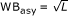that holds when M L. In evaluating the asymptotic curve, we assume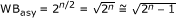.

For the smallest values of the span n, m-sequences and De Bruijn sequences show the lowest values of the bound; when n increases, De Bruijn sequences exhibit performance comparable to Gold and Kasami large set sequences. As shown, the asymptotic curve is well approached by the De Bruijn sequences, even for small values of n, thanks to the double exponential growth of M with n. As long as the value of the span n increases, the De Bruijn sequences show a better adherence to the Welch bound than the other families of spreading codes considered for comparison. Detailed values assumed by the bound for each family of sequences and for n = 3 and n = 10 are reported in Table 2.

### Auto- and cross-correlation properties of De Bruijn sequences

Any set of binary De Bruijn sequences of span n includes M/2 different sequences, and their corresponding complementary ones; so, in the set n = 5 we have 1,024 different sequences, and 1,024 complementary sequences. Table 3 provides a description of the statistical properties of the auto-correlation functions for the sequences included in this set; as shown, from the whole family of sequences, two subsets are extracted, corresponding to different thresholds on the maximum absolute value of the auto-correlation sidelobes (i.e., for shift τ ≠ 0). Low sidelobes in the auto-correlation functions of the CDMA spreading sequences allow a better synchronization at the receiver, so we select two subsets, Φ4 that contains 12 sequences, for which the maximum absolute value of the auto-correlation sidelobes is 4, and Φ8 that includes 784 sequences, for which the maximum absolute value of the auto-correlation sidelobes is 8. As expected, all the sequences in any set have an average auto-correlation equal to 0.

The cross-correlation function computed between two complementary De Bruijn sequences always shows a negative peak value of - 2n, for a shift τ = 0. As a consequence, given the DS-CDMA context of application, it is necessary to avoid the presence of complementary sequences in the set from which spreading codes are chosen. This constraint will limit our analysis to 1,024 sequences of span n = 5. Table 4 describes the statistical properties of the cross-correlation functions computed over 1,024 De Bruijn sequences of span 5 that are divided into different subsets by setting different thresholds on the maximum absolute value of the cross-correlation peak. The analysis performed on the cross-correlation properties shows that the two sequences extracted from the half set, for which the cross-correlation absolute peak value is 8, are also the two optimum sequences for auto-correlation. We also observe that in the subset Φ4, when the threshold on the maximum absolute value of the cross-correlation peak decreases, the statistical figures evaluated increase. It means that if we try to extract sequences having low auto-correlation sidelobes, like those in Φ4, we cannot simultaneously reduce the cross-correlation peak and sidelobes values. If we want a limited cross-correlation peak, we must accept higher sidelobes, and viceversa. As a further remark, we may say that high values of the cross-correlation functions (i.e., greater than 12) are sporadically obtained; however, when these values appear, and the cross-correlation between two sequences gets higher than 20, the effects on the DS-CDMA system performance are disruptive.

Results similar to those presented in Table 3 have been derived also for a partial set of De Bruijn sequences of span 6. The generation of span 6 De Bruijn sequences is performed by resorting to the "tree approach" under development. In a first round, the generated paths are pruned every 8 steps; by this way, we limit the generation to a partial set of 268,510 sequences. Among them, we select those sequences for which the maximum absolute value of the auto-correlation sidelobes does not exceed 8, and we obtain 127 sequences. These are further selected into a subset of 15 sequences, for which the maximum cross-correlation equals 24, and into a subset of 34 sequences, for which the maximum cross-correlation equals 28. It is worth noting that even when limiting the subset of sequences to those having a maximum absolute value of the auto-correlation sidelobes equal to 8, we still get 127 different sequences among which we can select the required spreading codes for the DS-CDMA system.

A similar approach is applied to the sequences generated by pruning the partial paths every 6 steps. A smaller set is obtained, including 4,749 sequences, among which we select 736 sequences having a maximum absolute value of the auto-correlation sidelobes equal to 12. From this subset, we further select 7 sequences with a maximum cross-correlation peak equal to 24, and 18 sequences with a maximum cross-correlation peak of 28. The properties of the sequences obtained are described in Tables 5 and 6.

Considering the family of span 5 De Bruijn sequences that we can generate exhaustively, once obtained the subset Φ4 including sequences with favorable cross-correlation functions, we tested the possibility of adopting them as spreading codes in the downlink and uplink sections of a DS-CDMA system, for different numbers of users. We computed the average error probability at the output of a correlator receiver of the i th user, in a gaussian channel affected by multipath, according to the Channel A indoor and outdoor-to-indoor test environments specified in . The performance provided by the adoption of De Bruijn sequences are compared to those obtainable by adopting OVSF sequences in the dowlink section, Gold sequences in the uplink section, and to the ideal behavior of the system (no interference). Some results are also provided, related to the outdoor test environment only, for sequences of span n = 6.

### Downlink section, span n = 5

Simulations in the downlink section of the CDMA system are performed by comparing De Bruijn and OVSF sequences of length 32, in the case of 2, 3, and 4 active users. De Bruijn sequences belong to the set Φ4 that includes 12 pairwise complementary sequences: 6 sequences are chosen, by excluding the corresponding complementary ones, so that they may result orthogonal with respect to the corresponding cross-correlation. At the same time, 32 OVSF sequences are generated, and the average performance computed over all the possible subsets of 4 sequences obtainable from the whole set.

Simulation results are shown in Figures 4 and 5, for the indoor and outdoor Channel A test environments, respectively. The average probability of error is estimated, for the E b /N0 parameter ranging from 6 to 14 dB or 12 dB, and for a number of active users equal to 2, 3 and 4.

As a general remark, we may observe that De Bruijn sequences generally perform slightly better than OVSF sequences, thanks to their more favorable autocorrelation profiles, with respect to OVSF codes. The improvement brought by the adoption of De Bruijn sequences is more evident for higher values of the E b /N0 parameter.

### Uplink section, span n = 5

In the uplink section of the CDMA system, we compare De Bruijn sequences of length 32 and Gold sequences of length 31, in the case of 2, 3, and 4 active users. De Bruijn sequences are selected in the set Φ8 that includes 7 sequences showing a maximum absolute value of the cross-correlation equal to 12. The performance is averaged over all the possible selections of 2, 3, and 4 sequences in the whole set. In a similar way, we also test the performance provided by the set of 33 Gold sequences, by averaging the results obtained by different choices of 4, 3, and 2 spreading codes.

Figures 6 and 7 show the estimated behavior, in the indoor and outdoor Channel A test environments, respectively. Again, the average probability of error is estimated for the E b /N0 parameter ranging from 6 to 14 dB or 12 dB.

It is evident that in all the situations considered, Gold codes perform better than De Bruijn ones, even if the differences in the average probability of error are not so significant. We can say that De Bruijn sequences are comparable to OVSF codes, whereas they do not perform so good with respect to Gold sequences. The last comparison we provide refers to the outdoor test environment only, for span n = 6.

As a final evaluation, we consider span 6 sequences, i.e., OVSF sequences of length 64, Gold codes of length 63, and De Bruijn sequences of length 64 belonging to the subset Φ8 in Table 5 made of sequences showing a maximum value of the cross-correlation equal to 28. We test their performance in the outdoor test environment only, either in the downlink or in the uplink sections. Similar to the previous test, we compare De Bruijn sequences to Gold codes in the uplink section, and to the OVSF codes in the downlink section, and consider the case of four users active in the system. Figure 8 shows the average error probability for different values of the E b /N0 parameter. It is confirmed that Gold codes perform better than De Bruijn ones, even for increased span, whereas De Bruijn sequences are better than OVSF codes in the downlink section.

## Conclusion

This article presented some results about the application of binary De Bruijn sequences in DS-CDMA systems, as user spreading codes. Binary De Bruijn sequences feature great cardinality of the available sequence sets, even for small values of the span parameter, and may consequently allow the definition of proper selection criteria, based on thresholds applied on the auto- and cross-correlation profiles, though preserving a great number of available codes. The performance provided by De Bruijn sequences have been compared to those obtained by more consolidated solutions, relying on the use of m-sequences, Gold, and OVSF sequences as spreading codes. From simulations, it is evident that De Bruijn codes show a rather similar behavior to the code sets traditionally considered, and designed ad hoc to provide good CDMA performance. Consequently, the results discussed in this article encourage further studies and analyses, to extensively test the applicability of De Bruijn sequences in multi-user contexts, even by resorting to longer codes, that, however, require more sophisticated generation techniques. At the same time, a thorough investigation of the sequences correlation properties is fundamental, to design suitable selection criteria for each specific application scenario.

## Abbreviations

CDMA:

code division multiple access

DS:

direct sequence

LFSRs:

Linear Feedback Shift Registers.

## References

1. Pursley MB: Performance evaluation for phase-coded spread spectrum multiple-access communication--part I: system analysis. IEEE Trans Commun 1977, COM-25(8):795-799.

2. Dinan EH, Jabbari B: Spreading Codes for Direct Sequence CDMA and Wideband CDMA Cellular Networks. IEEE Commun Mag 1998, 36(9):48-54. 10.1109/35.714616

3. Haykin S: Communication Systems. 4th edition. Wiley, New York; 2001.

4. Sarwate DV, Pursley MB: Crosscorrelation properties of pseudorandom and related sequences. Proc IEEE 1980, 68(05):593-619.

5. Pursley MB, Sarwate DV: Performance evaluation for phase-coded spread spectrum multiple-access communication-part ii: code sequence analysis. IEEE Trans Commun 1977, COM-25(8):800-802.

6. Minn T, Siu K-Y: Dynamic assignment of orthogonal variable-spreading-factor codes in W-CDMA. IEEE J Sel Areas Commun 2000, 18(8):1429-1440. 10.1109/49.864008

7. De Bruijn N: A combinatorial problem. Proc Ned Akad Wet 1946, 49: 758-764.

8. Mayhew GL: Clues to the hidden nature of de Bruijn sequences. Comput Math Appl 2000, 39: 57-65.

9. Fredricksen H: A survey of full length nonlinear shift register cycle algorithms. SIAM Rev 1982, (24):195-221.

10. Mitchell CJ, Etzion T, Paterson KG: A method for constructing decodable de Bruijn sequences. IEEE Trans Inf Theory 1996, 42(5):1472-1478. 10.1109/18.532887

11. Annexstein FS: Generating De Bruijn sequences: an efficient implementation. IEEE Trans Comput 1997, 46(2):198-200. 10.1109/12.565596

12. Andrenacci S, Gambi E, Spinsante S: Preliminary results on the adoption of De Bruijn binary sequences in DS-CDMA Systems. In Multiple Access Communications, Proc. of MACOM 2010. Volume 6235. LNCS, Springer, Berlin, Heidelberg; 2010:58-69. 10.1007/978-3-642-15428-7_6

13. Etzion T, Lempel A: Algorithms for the generation of full-length shift-register sequences. IEEE Trans Inform Theory 1984, IT-30(3):480-484.

14. Welch LR: Lower bounds on the maximum cross-correlation of signals. IEEE Trans Inform Theory 1974, (IT-20):397-399.

15. Recommendation ITU-R M.1225: "Guidelines for Evaluation of Radio Transmission Technologies for IMT-2000". International Telecommunication Union 1997.

16. Zhaozhi Z, Wende C: Correlation properties of De Bruijn sequences. Syst Sci Math Sci Acad Sinica 1989, 2(2):170-183.

17. Zhang W, Liu S, Huang H: An efficient implementation algorithm for generating de Bruijn sequences. J Comput Stand Interfaces 2009, 31(6):1190-1191. 10.1016/j.csi.2008.12.001

18. Golomb SW: Shift Register Sequences. Aegean Park Press, Laguna Hills; 1981.

## Author information

Authors

### Corresponding author

Correspondence to Susanna Spinsante.

### Competing interests

The authors declare that they have no competing interests.

## Authors’ original submitted files for images

Below are the links to the authors’ original submitted files for images.

## Rights and permissions

Reprints and Permissions

Spinsante, S., Andrenacci, S. & Gambi, E. Binary De Bruijn sequences for DS-CDMA systems: analysis and results. J Wireless Com Network 2011, 4 (2011). https://doi.org/10.1186/1687-1499-2011-4

• Accepted:

• Published:

• DOI: https://doi.org/10.1186/1687-1499-2011-4

### Keywords